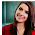# Computer System Architecture Set 10

### Questions 91 to 100

91.
Which of the following Addressing Modes specifies a register which contains the memory address of the operand?
 (a) Indirect Addressing Mode (b) Register Addressing Mode (c) Register Indirect Addressing Mode (d) Index Addressing  Mode (e) Base Address Register Addressing Mode.
92.
Identify the term, which indicate a set of Logical Addresses.
 (a) Memory space (b) Disk space (c) Address space (d) Location (e) Page frame.
93.
Which of the following is false related to Stack?
 (a) Stack Pointer points to the top most element of the stack (b) Only PUSH and POP operations are applicable (c) Implements FIFO (d) Useful for nested loops, subroutine calls etc (e) Efficient for arithmetic expression evaluation.
94.
Which of the following is the method in which the unit receiving the data responds with another control signal?
 (a) Timing sequence (b) Synchronous (c) Strobe (d) Short message service (e) Handshaking.
95.
What is the page replacement algorithm in which there is a replacement of a page, which will not be used for the longest period of time?
 (a) MFU (b) LRU (c) OPT (d) FIFO (e) LFU.
96.
Of the following, what is an input device for which finger shows the cursor movement?
 (a) Mouse (b) Scanner (c) Microphone (d) Trackball (e) Glide pad.
97.
Consider the following unsigned  decimal numbers:
i.       6543.
ii.      4444.
What is the result after subtracting (i) by taking the 10's complement of (ii)?
 (a) 2098 (b) 2099 (c) 2096 (d) 2097 (e) 2095
98.
What is the octal equivalent of given binary number?
011001
 (a) 32 (b) 31 (c) 34 (d) 33 (e) 35
99.
Which of the following describes the Mnemonic SPA?
 (a) Skip If (Address Resister) Positive (b) Skip If (Accumulator) Positive (c) Skip If (Adder-Subtractor) Positive (d) Skip If (Associative Mapping) Positive (e) Skip If (Assembler) Positive.
100.
Which of the following is correct related to the circuit diagrams?
 (a) Sequential circuit is an interconnection of only logic gates (b) Sequential circuit is an interconnection of only flip flops (c) Combinational circuit is an interconnection of  logic gates (d) Combinational circuit is an interconnection of flip flops (e) Part of a combinational circuit is a sequential circuit.

#### Answers

 91 Answer :       (c) Reason:  Addressing mode that specifies a register which contains the memory address of the operand is  Register Indirect Addressing Mode. 92 Answer :       (c) Reason:  Address space indicate a set of Logical Addresses. 93 Answer :       (c) Reason:  Stack does not Implement FIFO but implements LIFO. 94 Answer :       (e) Reason:  Handshaking is the method in which the unit receiving the data responds with another control signal. 95 Answer :       (c) Reason:  The page replacement algorithm in which there is a replacement of a page, which will not be used for the longest period of time is OPT. 96 Answer :       (e) Reason:  The input device for which finger shows the cursor movement is Glide pad. 97 Answer :       (b) Reason:  6543- 4444= 2099 98 Answer :       (b) Reason:  The octal equivalent of  011001 =31. 99 Answer :       (b) Reason:  SPA stands for Skip If (Accumulator) Positive 100 Answer :       (c) Reason:  Combinational circuit is an interconnection of logic gates.

#### 2 comments :

1.It has been simply incredibly generous with you to provide openly what exactly many individuals would’ve marketed for an eBook to end up making some cash for their end, primarily given that you could have tried it in the event you wanted.

AWS Training in Bangalore

2.Hey, would you mind if I share your blog with my twitter group? There’s a lot of folks that I think would enjoy your content. Please let me know. Thank you.
AWS Training in Chennai
Data Science Training in Chennai
Python Training in Chennai
RPA Training in Chennai
Digital Marketing Training in Chennai